Square Wave Generator

This square wave generator is like the Schmitt trigger circuit in that the reference voltage for the comparator action depends on the output voltage. This circuit is also classified as an astable multivibrator.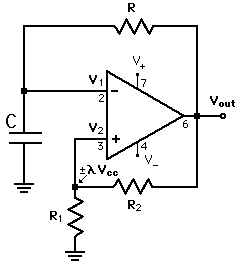Comparator Applications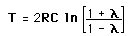Show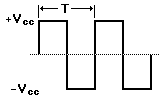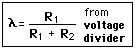Voltage Divider
 Examine Process
 Calculation
Index

Electronics concepts

Op-amp concepts

Reference
Simpson

 HyperPhysics*****Electricity and magnetism R Nave
Go Back

Period of Square Wave Generator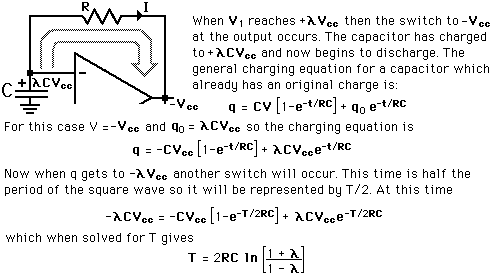More Detail Square wave generator circuit
Index

Electronics concepts

Op-amp concepts

Reference
Simpson

 HyperPhysics*****Electricity and magnetism R Nave
Go Back

Square Wave Period: Details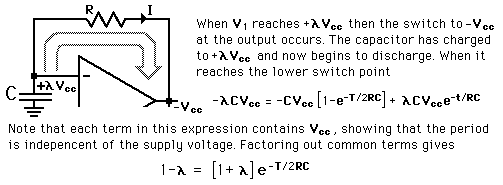Now taking the natural logarithm of both sides: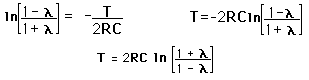Calculation Square wave generator circuit
Index

Electronics concepts

Op-amp concepts

Reference
Simpson

 HyperPhysics*****Electricity and magnetism R Nave
Go Back

Square Wave GeneratorShowDiscussion of circuit
For circuit parameters: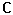=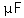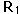=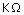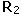=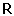=the period will be
 T = x 10^ s
and the frequency of the square wave is
 f = kHz = MHz

Note that even though the square wave generator swings the voltage output from plus to minus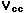, the frequency does not depend upon this supply voltage. If you supplied it with a variable voltage, you could freely change the amplitude without changing the frequency. You could then make it a variable frequency source by making either C or R variable.

 Show expressions
Index

Electronics concepts

Op-amp concepts

 HyperPhysics*****Electricity and magnetism R Nave
Go Back

Square Wave Generator Process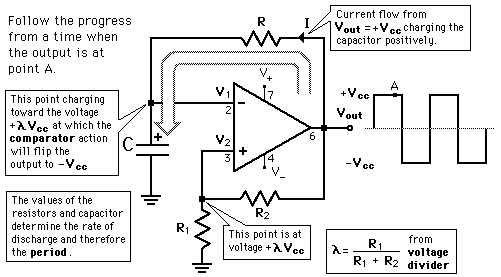Square Wave Generator Calculation Development of period expression
 Comparator Voltage Divider
Index

Electronics concepts

Op-amp concepts

Reference
Simpson

 HyperPhysics*****Electricity and magnetism R Nave
Go Back